Next: Pyramidal Algorithm Up: The discrete wavelet transform Previous: Multiresolution Analysis

## The à trous algorithm

The discrete approach of the wavelet transform can be done with the special version of the so-called à trous algorithm (with holes) [#holschn1<#14462,#shensa<#14463]. One assumes that the sampled data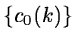are the scalar products at pixels k of the function f(x) with a scaling function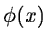which corresponds to a low pass filter.

The first filtering is then performed by a twice magnified scale leading to theset. The signal difference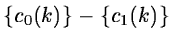contains the information between these two scales and is the discrete set associated with the wavelet transform corresponding to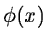. The associated wavelet is therefore.(14.29)

The distance between samples increasing by a factor 2 from the scale (i-1) (i > 0) to the next one, ci(k) is given by: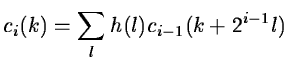(14.30)

and the discrete wavelet transform wi(k) by:

 wi(k) = ci-1(k) - ci(k) (14.31)

The coefficients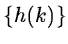derive from the scaling function: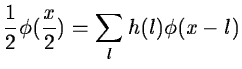(14.32)

The algorithm allowing one to rebuild the data frame is evident: the last smoothed array cnp is added to all the differences wi.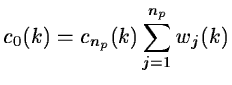(14.33)

If we choose the linear interpolation for the scaling function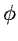(see figure 14.5):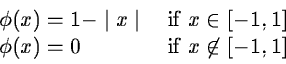we have: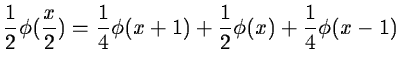(14.34)

c1 is obtained by: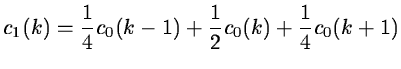(14.35)

and cj+1 is obtained from cj by: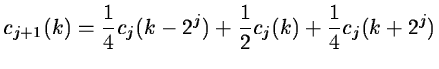(14.36)

The figure 14.6 shows the wavelet associated to the scaling function.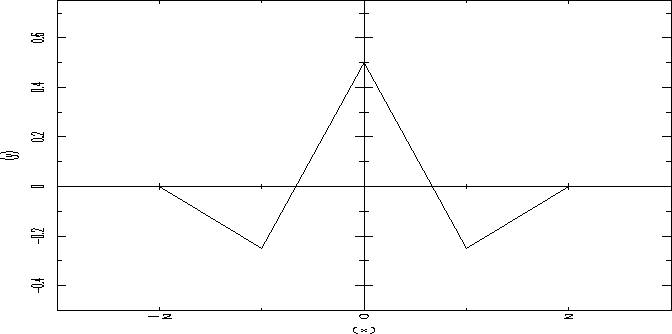The wavelet coefficients at the scale j are: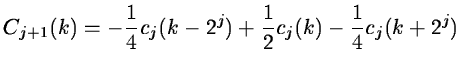(14.37)

The above à trous algorithm is easily extensible to the two dimensional space. This leads to a convolution with a mask ofpixels for the wavelet connected to linear interpolation. The coefficents of the mask are: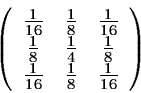At each scale j, we obtain a set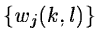(we will call it wavelet plane in the following), which has the same number of pixels as the image.

If we choose a B3-spline for the scaling function, the coefficients of the convolution mask in one dimension are (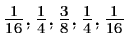), and in two dimensions: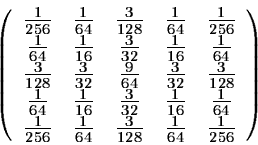Next: Pyramidal Algorithm Up: The discrete wavelet transform Previous: Multiresolution Analysis
Petra Nass
1999-06-15